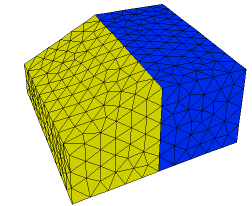﻿ Sample Problems > Usage > 3D_domains > tabular_surfaces

# tabular_surfaces

Navigation:  Sample Problems > Usage > 3D_domains >

# tabular_surfaces{ TABULAR_SURFACES.PDE

This problem demonstrates the use of tabular input and regional definition

for 3D extrusion surfaces.

The bottom surface of a brick is read from a table.

Note:  Tables by default use bilinear interpolation.  Mesh cell boundaries do NOT

automatically follow table boundaries, and sharp slope breaks in table data

can result in ragged surfaces.  You should always make surface tables dense

enough to avoid sharp breaks, or put domain boundaries or features along

breaks in the table slope.  You should also specify mesh density controls

sufficiently dense to resolve table features.

The top surface is defined by different functions in two regions.

Note: the regional surface definitions must coincide at the region boundaries

where they meet.  Surfaces must be continuous and contain no jumps.

 }   title 'tabular surface definition'   coordinates    cartesian3   variables    Tp   definitions    long = 1    wide = 1    K  = 1    Q = 10*exp(-x^2-y^2-z^2)     { read the table file for surface 1 definition: }    z1 = table('surf.tbl')     { use regional parameters for surface 2 definition: }    z2               initial values    Tp = 0.   equations    Tp:  div(k*grad(Tp)) + Q = 0extrusion z = z1,z2     { define two surfaces from previously declared parameters }

boundaries

surface 1 value(Tp)=0

surface 2 value(Tp)=0

Region 1

z2 = 1           { default surface 2 over total domain }

start(-wide,-wide)

value(Tp) = 0

line to (wide,-wide)

to (wide,wide)

to (-wide,wide)

to close

Region 2

z2 = 1 + x/2     { override surface 2 definition in region 2 }

start(-wide,-wide)

line to (0,-wide)

to (0,wide)

to (-wide,wide)

to close

monitors

grid(x,z) on y=0

plots

grid(x,z) on y=0

contour(Tp) on y=0 as "ZX Temp"

contour(Tp) on x=0 as "YZ Temp"

end